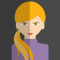## Using iterative function print the given number in reverse order in C languagePublished on 05-Mar-2021 10:23:25
ProblemHow to print the given in reverse order with the help of iterative function i.e., while loop using C programming language?SolutionSo far, we had seen how to reverse the string using string function and without string function, now let’s see how to reverse a number without using predefined function −AlgorithmInput ... Read More

## Printing the numbers in reverse order using Division and modulo operators using CPublished on 05-Mar-2021 10:06:20
ProblemHow to print the given two-digit number in reverse order with the help of division and Modulo operator using C programming language?SolutionSo far, we had seen how to reverse the string using string function and without string function. Now let’s see how to reverse the two-digit number without using the ... Read More

## Differentiate the modulo and division by using C Programming language?Published on 05-Mar-2021 10:03:54
Modulo − Represents as % operator. And gives the value of the remainder of an integer division.Division − represents as / operator. And gives the value of the quotient of a division.Program 1#include<stdio.h> int main(){    int a, b, c;    printf("enter a, b, c values:");    scanf("%d%d%d, &a, &b, &c);   ... Read More

## Write a C program to perform 3X3 matrix operationsPublished on 05-Mar-2021 09:59:17
ProblemEnter any 9 numbers at runtime and add the numbers in a row, column, and diagonal wise by using C Programming languageAlgorithmStep 1: Declare 9 variables Step 2: enter 9 numbers at runtime Step 3: store the numbers in 3 X 3 matrix form         //x y ... Read More

## Write a C program of library management system using switch casePublished on 05-Mar-2021 09:57:05
ProblemHow to store the books-related information of library using C programming.AlgorithmStep 1: Declare a structure which holds data members Step 2: declare variables which are used for loop Step 3: use switch case to work on each module Step 4: case 1- for Adding book information         ... Read More

## Write a C program for electing a candidate in Elections by calling functions using Switch casePublished on 05-Mar-2021 08:49:06
ProblemHow to cast vote, count, and display the votes for each candidate that participates in elections using C language?SolutionLet’s consider three persons who participated in elections. Here we need to write a code for the following −Cast vote − Selecting a candidate by pressing the cast voteFind vote count − ... Read More

## How to align the output using justificationsin C language?Published on 05-Mar-2021 08:43:18
By using justifications in printf statement we can arrange the data in any format.Right JustificationTo implement the right justification, insert a minus sign before the width value in the %s character.printf("%-15s", text);Program 1Let’s take an example to print data in row and column-wise with the help of justification. Live Demo#include<stdio.h> int main(){ ... Read More

## Write C program to calculate balance instalmentPublished on 05-Mar-2021 08:39:45
ProblemWrite a C program to calculate a balance installment that is to be paid after every month for a particular loan amount (with interest).SolutionFollowing is the formula to calculate interest when the loan amount is given −i=loanamt * ((interest/100)/12);Following calculation gives amount with interest −i=i+loanamt; firstmon=i-monthlypayment; //first month payment with ... Read More

## C program on calculating the amount with tax using assignment operatorPublished on 05-Mar-2021 08:36:52
ProblemWrite a C Program to enter the amount in dollars and then display the amount by adding 18% as tax.SolutionLet’s consider the restaurant person adding 18% tax to every bill of the customer.The logic applied to calculate tax is −value=(money + (money * 0.18));The money should be multiplied by 18% ... Read More

## How to calculate the volume of a sphere using C programming language?Published on 05-Mar-2021 08:33:38
The volume of sphere is nothing but the capacity of the shape.Volume of a sphere formula is −$$V\:=\:\frac{4}{3}\Pi\:r^{3}$$AlgorithmStep 1: Enter radius of sphere at runtime Step 2: Apply the formula to variable         Volume=(4/3)*3.14*rad*rad*rad Step 3: print the volume Step 4: stopProgram 1 Live Demo#include<stdio.h> int main(){   ... Read More